# HSSlive: Plus One & Plus Two Notes & Solutions for Kerala State Board

## AP Board Class 7 Maths Chapter 10 Algebraic Expressions Ex 1 Textbook Solutions PDF: Download Andhra Pradesh Board STD 7th Maths Chapter 10 Algebraic Expressions Ex 1 Book AnswersAP Board Class 7 Maths Chapter 10 Algebraic Expressions Ex 1 Textbook Solutions PDF: Download Andhra Pradesh Board STD 7th Maths Chapter 10 Algebraic Expressions Ex 1 Book Answers

## Andhra Pradesh State Board Class 7th Maths Chapter 10 Algebraic Expressions Ex 1 Books Solutions

 Board AP Board Materials Textbook Solutions/Guide Format DOC/PDF Class 7th Subject Maths Chapters Maths Chapter 10 Algebraic Expressions Ex 1 Provider Hsslive

2. Click on the Andhra Pradesh Board Class 7th Maths Chapter 10 Algebraic Expressions Ex 1 Answers.
3. Look for your Andhra Pradesh Board STD 7th Maths Chapter 10 Algebraic Expressions Ex 1 Textbooks PDF.
4. Now download or read the Andhra Pradesh Board Class 7th Maths Chapter 10 Algebraic Expressions Ex 1 Textbook Solutions for PDF Free.

## AP Board Class 7th Maths Chapter 10 Algebraic Expressions Ex 1 Textbooks Solutions with Answer PDF Download

Find below the list of all AP Board Class 7th Maths Chapter 10 Algebraic Expressions Ex 1 Textbook Solutions for PDF’s for you to download and prepare for the upcoming exams:

Question 1.
Find the rule which gives the number of matchsticks required to make the following patterns
(i) A pattern of letter ‘H’
(ii) A pattern of letter ‘V’
Solution:
i) A pattern of letter ‘H’
The letter ‘H’ is formed with 3 matchsticks.
∴ Its pattern should be 3, 6, 9, 12 (or)
3 × 1, 3 × 2, 3 × 3, 3 × 4 ……………….. 3 x n
∴ The required pattern is 3 × n = 3n

ii) A pattern of letter ‘V’
The letter ‘V’ is formed with 2 matchsticks.
∴ Its pattern should be 2, 4. 6. 8 (or)
2 × 1, 2 × 2, 2 × 3 …………………2 × n
∴ The required pattern is 2 × n = 2n

Question 2.
Given below is a pattern made from coloured tiles and white tiles.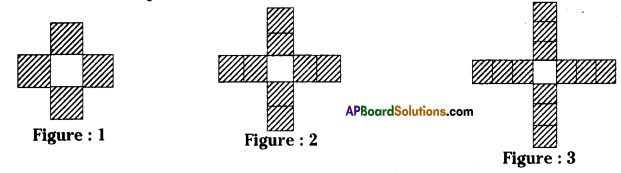(i) Draw the next two figures in the pattern above.
(ii) Fill the table given below and express the pattern in the form of an algebraic expression.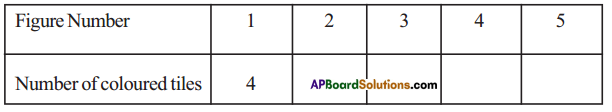(iii) Fill the table given below and express the pattern in the form of an algebraic expression.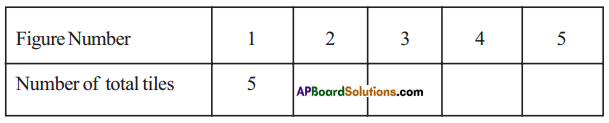Solution: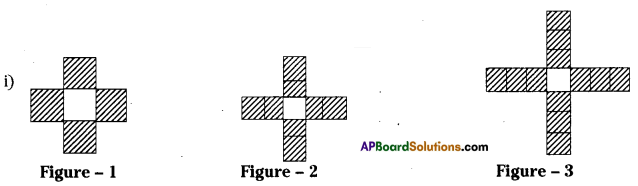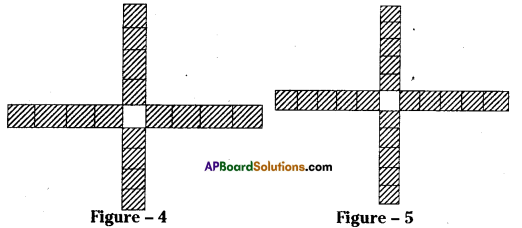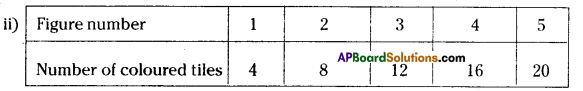∴ The required algebraic expression is “4”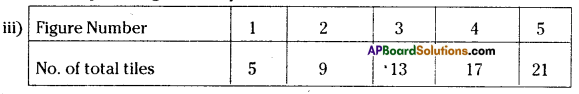∴ The required algebraic expression is “4+1”
If n = 1 ⇒ 4n + 1 = 4(1) + 1 = 5
n = 2 ⇒ 4 × 2 + 1 = 8 + 1 = 9
n = 3 ⇒ 4 × 3 + 1 = 12 + 1 =13 ……………….. soon.

Question 3.
Write the following statements using variables, constants and arithmetic operations.
(i) 6 more than p
(ii) ‘x’ is reduced by 4
(iii) 8 subtracted from y
(iv) q multiplied by ‘-5’
(v) y divided by 4
(vi) One-fourth of the product of ’p’ and ‘q’
(vii) 5 added to the three times of ’z’
(viii) x multiplied by 5 and added to ‘10’
(ix) 5 subtracted from two times of ‘y’
(x) y multiplied by 10 and added to 13
Solution:

 Sentence Algebraic expression 1. 6 more than p p + 6 2. x is reduced by 4 x – 4 3. 8 subtracted from y y – 8 4. q multiplied by ‘-5’ -5q 5. y divided by 4 𝑦4 6. One-fourth of the product of ‘p’ and ‘q’ pq4 7. 5 added to the three times of z 3z + 5 8. ‘x’ multiplied by 5 and added to 10 5x + 10 9. 5 subtracted from two times of ‘y’ 2y – 5 10. y multiplied by 10 and added to 13 10y + 13

Question 4.
Write the following expressions in Statements.
(i) x + 3
(ii) y – 7
(iii) 10l
(iv) 𝑥5
(v) 3m + 11
(vi) 2y – 5
Solution:
Expression Statements

 Expression Statements i) x + 3 x is added to 3 ii) y – 7 7 is subtracted from y. iii) 10l l is multiplied by 10. iv) x5 x is divided by 5 v) 3m + 11 m is multiplied by 3 and added to 11 vi) 2y – 5 y is multiplied by 2 and 5 ¡s subtracted from the product

Question 5.
Some situations are given below. State the number in situations is a variable or constant?
Example: Our age – its value keeps on changing so it is an example of a variable quantity.
(i) The number of days in the month of January
(ii) The temperature of a day
(iv) Height of the growing plant
Solution:
i) No. of days ¡n the month of January are fixed in every year. So, “Number of days” is a constant.
ii) The temperature of a day changes every minute. So (temperature) it ¡s a variable.
iii) The length of the classroom is fixed. So it is a constant.
iv) The height of a growing plant changes in every month. So it is a variable.

## Andhra Pradesh Board Class 7th Maths Chapter 10 Algebraic Expressions Ex 1 Textbooks for Exam Preparations

Andhra Pradesh Board Class 7th Maths Chapter 10 Algebraic Expressions Ex 1 Textbook Solutions can be of great help in your Andhra Pradesh Board Class 7th Maths Chapter 10 Algebraic Expressions Ex 1 exam preparation. The AP Board STD 7th Maths Chapter 10 Algebraic Expressions Ex 1 Textbooks study material, used with the English medium textbooks, can help you complete the entire Class 7th Maths Chapter 10 Algebraic Expressions Ex 1 Books State Board syllabus with maximum efficiency.

## FAQs Regarding Andhra Pradesh Board Class 7th Maths Chapter 10 Algebraic Expressions Ex 1 Textbook Solutions

#### Can we get a Andhra Pradesh State Board Book PDF for all Classes?

Yes you can get Andhra Pradesh Board Text Book PDF for all classes using the links provided in the above article.

## Important Terms

Andhra Pradesh Board Class 7th Maths Chapter 10 Algebraic Expressions Ex 1, AP Board Class 7th Maths Chapter 10 Algebraic Expressions Ex 1 Textbooks, Andhra Pradesh State Board Class 7th Maths Chapter 10 Algebraic Expressions Ex 1, Andhra Pradesh State Board Class 7th Maths Chapter 10 Algebraic Expressions Ex 1 Textbook solutions, AP Board Class 7th Maths Chapter 10 Algebraic Expressions Ex 1 Textbooks Solutions, Andhra Pradesh Board STD 7th Maths Chapter 10 Algebraic Expressions Ex 1, AP Board STD 7th Maths Chapter 10 Algebraic Expressions Ex 1 Textbooks, Andhra Pradesh State Board STD 7th Maths Chapter 10 Algebraic Expressions Ex 1, Andhra Pradesh State Board STD 7th Maths Chapter 10 Algebraic Expressions Ex 1 Textbook solutions, AP Board STD 7th Maths Chapter 10 Algebraic Expressions Ex 1 Textbooks Solutions,
Share: